## Saturday, 30 November 2013

### Basic Graph - plot()

R has increasingly more packages that offer more sophisticated data visualisation techniques and functions. However, the basic graphic function (plot()) still provides a good range of types and styles of graphs that would be sufficient for most users, albeit coding may be more cumbersome than the ones offered by packages, especially for more sophisticated graphs. The below function displays some of the built-in sample graphs drawn by basic graph command.
demo(graphics)
As with drawing graphs by hand, plot() function needs the user to assign x and y coordinates, either as respective vectors or as columns within data frame. R will examine the values of assigned x & y coordinates, and will try to make a guess for the most appropriate type of graph. The below are some of the examples of different ways of writing plot() function and the way R determines for the most appropriate graph types.
• When x, y coordinates are not assigned, and the data frame has only 2 numerical variables.
plot(cars)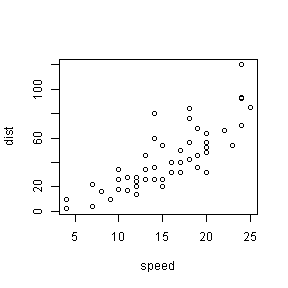• When x, y coordinates are assigned as a formula of one categorical and one numerical variables from a data frame.
plot(breaks ~ tension, warpbreaks)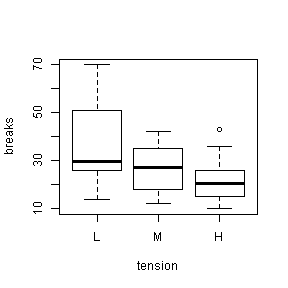• When x, y coordinates are not assigned, but the data frame has more than two variables.
plot(LifeCycleSavings)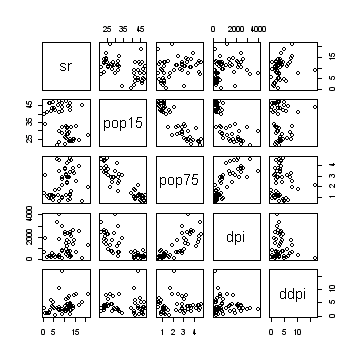The below series of codes demonstrate how each of the graphic parameters for plot() affects display of the graph.
1. This is the base graph with default values for all graphic parameters.

x <- c(0:100)
y <- 1/x
plot(x, y)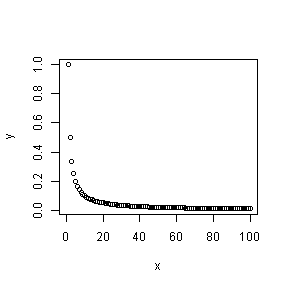2. 'pch' changes the style of the plots. It can be assigned either a number between 0-25 or an actual symbol.

plot(x, y, pch="+")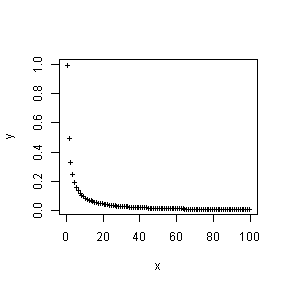plot(x, y, pch=25)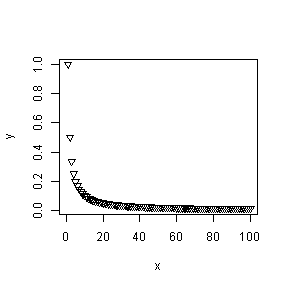3. 'cex' stands for 'character expansion', and it can be assigned a numeric value, including decimal places, to change the size of plot.

plot(x, y, pch=25, cex=2)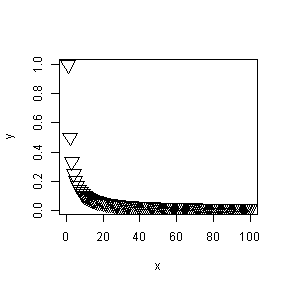4. 'type' determines the type of graph. The following are the possible options:
- "p" for points
- "l" for lines
- "b" for both
- "c" for the lines part alone of "b"
- "o" for both ‘overplotted’
- "h" for ‘histogram’ like (or ‘high-density’) vertical lines
- "s" for stair steps
- "S" for other steps
- "n" for no plotting

plot(x, y, type="h")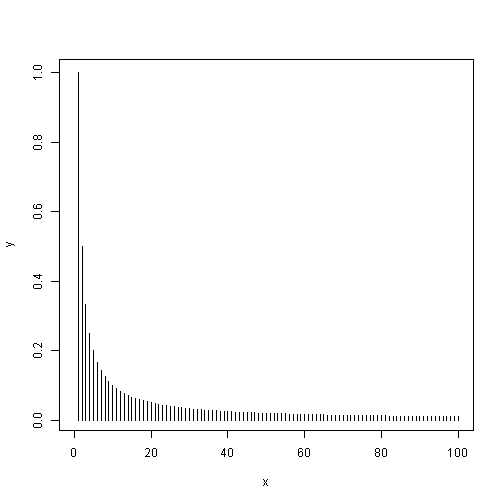5. 'lty' changes the style of lines. 1 is a solid line and 2 and above represent dotted lines of different styles. plot(x, y, type="l", lty=1)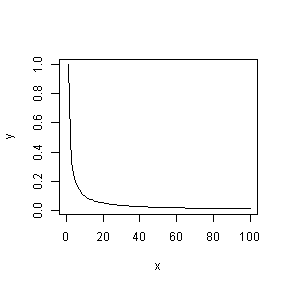plot(x, y, type="l", lty=3)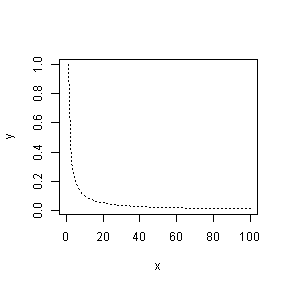6. 'lwd' changes the width of the line plot(x, y, type = "l", lty = 1, lwd = 3)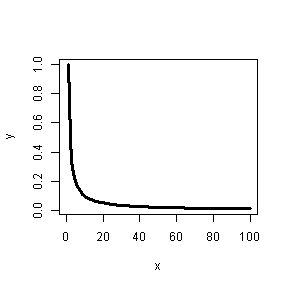7. 'col' changes the colour, and can be assigned a numerical value or a name of the actual colour. plot(x, y, type = "p", pch = 16, cex = 1.5, col = 2)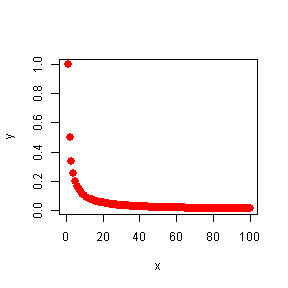plot(x, y, type = "l", lty = 1, lwd = 2, col = 3)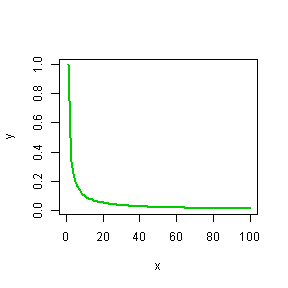plot(x, y, type = "l", lty = 3, lwd = 1, col = "blue")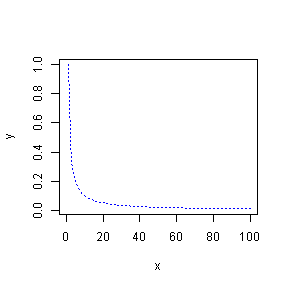8. For inserting title and subtitle, use 'main' and 'sub', respectively.

plot(x, y, type = "l", lty = 1, lwd = 3, col = 2, main = "Test", sub = "Hyperbola")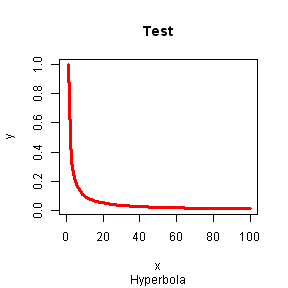9. For inserting axis labels, 'xlab' and 'ylab' inserts labels against x-axis and y-axis, respectively.

plot(x, y, type = "l", lty = 1, lwd = 3, col = 2, xlab = "X", ylab = "1/X", main = "Test", sub = "Hyperbola")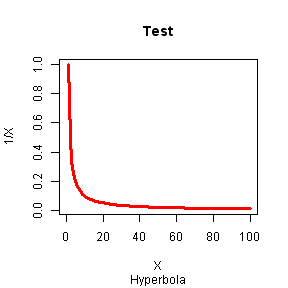10. 'col.main' and 'col.sub' changes colour of main title and subtitle, respectively.

plot(x, y, type = "l", lty = 1, lwd = 3, col = 2, col.main = 3, col.sub = 6, xlab = "X", ylab = "1/X", main = "Test", sub = "Hyperbola")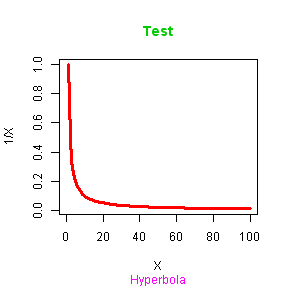11. 'col.lab' and 'col.axis' changes colour of axis labels and axis unit labels, respectively.

plot(x, y, type = "l", lty = 1, lwd = 3, col = 2, col.axis = 4, col.lab = 5, col.main = 3, col.sub = 6, xlab = "X", ylab = "1/X", main = "Test", sub = "Hyperbola")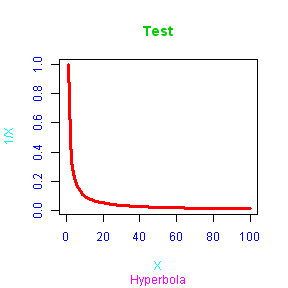12. When 'cex' is used as a prefix to certain parameters, it changes the size of the corresponding outputs. 'cex.main' changes size of title; 'cex.sub' changes size of subtitle; 'cex.lab' changes size of axis labels, and 'cex.axis' changes size of axis unit labels.

plot(x, y, type = "l", lty = 1, lwd = 3, col = 2, col.axis = 4, col.lab = 5, col.main = 3, col.sub = 6, cex.axis = 0.6, cex.main = 1.5, cex.sub = 1, cex.lab = 0.8, xlab = "X", ylab = "1/X", main = "Test", sub = "Hyperbola")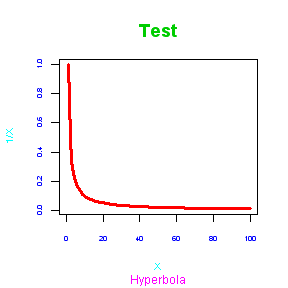13. The fonts of labels can be changed with the parameter 'font' or 'font....' as below.

plot(x, y, type = "l", lty = 1, lwd = 3, col = 2, col.axis = 4, col.lab = 5, col.main = 3, col.sub = 6, cex.axis = 0.6, cex.main = 1.5, cex.sub = 1, cex.lab = 0.8, xlab = "X", ylab = "1/X", main = "Test", sub = "Hyperbola", font = 2, font.axis = 2, font.lab = 1, font.main = 4, font.sub = 3)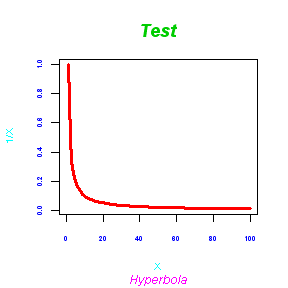14. 'adj' changes text alignment for labels. '0' is left aligned, '1' is right aligned and 0.5 is centre aligned. Other values between 0 and 1 represents the alignment in reference to the mentioned positions.

plot(x, y, type = "l", lty = 1, lwd = 3, col = 2, col.axis = 4, col.lab = 5, col.main = 3, col.sub = 6, cex.axis = 0.6, cex.main = 1.5, cex.sub = 1, cex.lab = 0.8, xlab = "X", ylab = "1/X", main = "Test", sub = "Hyperbola", font = 2, font.axis = 2, font.lab = 1, font.main = 4, font.sub = 3, adj = 0.7)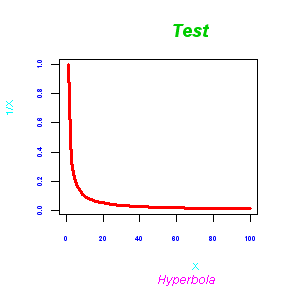15. 'lab' changes the format and size of axis unit labels. The parameter is usually assigned a vector that is written as (number of x-axis unit value, number of y-axis unit value, gap between unit values)

plot(x, y, type = "l", lty = 1, lwd = 3, col = 2, col.axis = 4, col.lab = 5, col.main = 3, col.sub = 6, cex.axis = 0.6, cex.main = 1.5, cex.sub = 1, cex.lab = 0.8, xlab = "X", ylab = "1/X", main = "Test", sub = "Hyperbola", font = 2, font.axis = 2, font.lab = 1, font.main = 4, font.sub = 3, adj = 0.7, lab = c(11, 3, 10))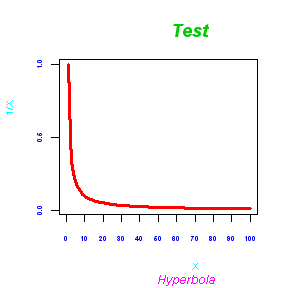16. 'las' changes orientation of the axis unit label. It is assigned a numeric value where '0' is parallel to axis, '1' is horizontal and '2' is vertical.

plot(x, y, type = "l", lty = 1, lwd = 3, col = 2, col.axis = 4, col.lab = 5, col.main = 3, col.sub = 6, cex.axis = 0.6, cex.main = 1.5, cex.sub = 1, cex.lab = 0.8, xlab = "X", ylab = "1/X", main = "Test", sub = "Hyperbola", font = 2, font.axis = 2, font.lab = 1, font.main = 4, font.sub = 3, adj = 0.7, lab = c(11, 3, 10), las = 2)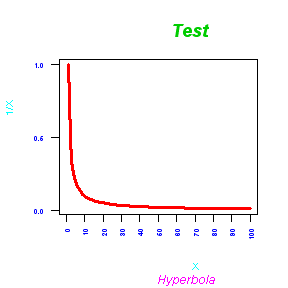17. 'mgp' changes the distances of axis label and axis unit label from the axis, as well as the distance between axis and side of the graph. It is assigned with a vector that is written as (distance between axis label and axis, distance between axis unit label and axis,distance between axis and side of the graph)

plot(x, y, type = "l", lty = 1, lwd = 3, col = 2, col.axis = 4, col.lab = 5, col.main = 3, col.sub = 6, cex.axis = 0.6, cex.main = 1.5, cex.sub = 1, cex.lab = 0.8, xlab = "X", ylab = "1/X", main = "Test", sub = "Hyperbola", font = 2, font.axis = 2, font.lab = 1, font.main = 4, font.sub = 3, adj = 0.7, lab = c(11, 3, 10), las = 2, mgp = c(3, 2, 1))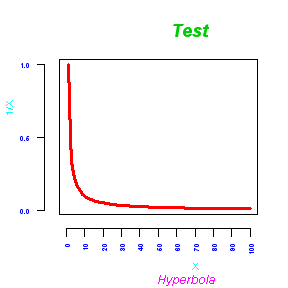18. 'tck' changes the length of tick marks on the axis. 'tck' is assigned a decimal point that is proportional to the plotting area when a grid line is given a value of 1. Positive number places tick marks inwards towards the graph, and negative number places the tick marks outwards.

plot(x, y, type = "l", lty = 1, lwd = 3, col = 2, col.axis = 4, col.lab = 5, col.main = 3, col.sub = 6, cex.axis = 0.6, cex.main = 1.5, cex.sub = 1, cex.lab = 0.8, xlab = "X", ylab = "1/X", main = "Test", sub = "Hyperbola", font = 2, font.axis = 2, font.lab = 1, font.main = 4, font.sub = 3, adj = 0.7, lab = c(11, 3, 10), las = 2, mgp = c(3, 2, 1), tck = 0.01)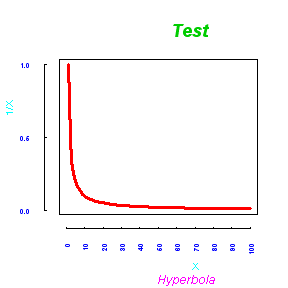19. R usually displays a graph with a bit of space at the extremes of x and y axes. This can be changed with 'xaxs' and 'yaxs'. The value of 'i' will leave no space and 'r' will leave space as per the default setting.

plot(x, y, type = "l", lty = 1, lwd = 3, col = 2, col.axis = 4, col.lab = 5, col.main = 3, col.sub = 6, cex.axis = 0.6, cex.main = 1.5, cex.sub = 1, cex.lab = 0.8, xlab = "X", ylab = "1/X", main = "Test", sub = "Hyperbola", font = 2, font.axis = 2, font.lab = 1, font.main = 4, font.sub = 3, adj = 0.7, lab = c(11, 3, 10), las = 2, mgp = c(3, 2, 1), tck = 0.01, xaxs = "i", yaxs = "r")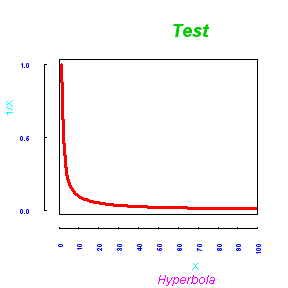20. 'mai' and 'mar' changes the margin of the graph. It is assigned as a vector that is written (bottom margin, left margin, top margion, right margin). 'mai' measures the margin by the unit value while 'mar' measures the margin by text line.

plot(x, y, type = "l", lty = 1, lwd = 3, col = 2, col.axis = 4, col.lab = 5, col.main = 3, col.sub = 6, cex.axis = 0.6, cex.main = 1.5, cex.sub = 1, cex.lab = 0.8, xlab = "X", ylab = "1/X", main = "Test", sub = "Hyperbola", font = 2, font.axis = 2, font.lab = 1, font.main = 4, font.sub = 3, adj = 0.7, lab = c(11, 3, 10), las = 2, mgp = c(3, 2, 1), tck = 0.01, xaxs = "i", yaxs = "r", mai = c(2, 1, 3, 0))